## Example Questions

### Example Question #1 : Monomials

Mike wants to sell candy bars for a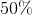profit. If he sells each bar for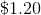, how much did each bar cost him?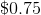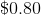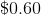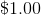Explanation:

In order to solve this problem, set up the following equation: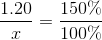Cross multiply: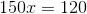Divide: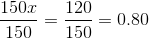The original cost of the of each candy bar is### Example Question #2 : Monomials

Choose the answer that is the simplest form of the following expression of monomial quotients: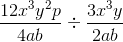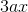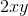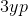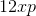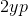Explanation:To divide monomial quotients, simply invert the divisor and multiply: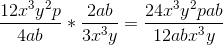Then, reduce: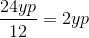### Example Question #3 : Monomials

Choose the answer that is the simplest form of the following expression of monomial quotients: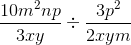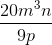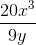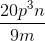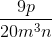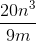Explanation:To find your answer, you have to invert the divisor and multiply across: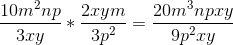Then, reduce: